# Kitchen

Kitchen roller has a diameter 70 mm and width of 359 mm. How many square millimeters roll on one turn?

Correct result:

S =  78948.2 mm2

#### Solution: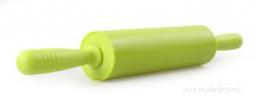We would be pleased if you find an error in the word problem, spelling mistakes, or inaccuracies and send it to us. Thank you!## Next similar math problems:

• Center traverseIt is true that the middle traverse bisects the triangle?
• Linear systemSolve this linear system (two linear equations with two unknowns): x+y =36 19x+22y=720When Bedrich is as old as Adam today, Adam will be 14 years old. When Adam will be as old as Bedrich, Bedrich was two years old today. How old are Adam and Bedrich today?
• Cylinder diameter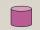The surface of the cylinder is 149 cm2. The cylinder height is 6 cm. What is the diameter of this cylinder?
• Fit ballWhat is the size of the surface of Gymball (FIT - ball) with a diameter of 65 cm?
• Liters od milkThe cylinder-shaped container contains 80 liters of milk. Milk level is 45 cm. How much milk will in the container, if level raise to height 72 cm?
• Cylinder surface areaVolume of a cylinder whose height is equal to the radius of the base is 678.5 dm3. Calculate its surface area.
• Surface of the cylinderCalculate the surface of the cylinder for which the shell area is Spl = 20 cm2 and the height v = 3.5 cm
• Concrete pipe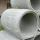How much will cost cover a 6 m long concrete pipe with an outer radius 1.5 m and inner radius 0.8 meters if 1 m2 paint costs 24 €.
• Cylinder - A&VThe cylinder has a volume 1287. The base has a radius 10. What is the area of surface of the cylinder?
• CylinderThe cylinder surface is 922 dm2, its height is equal to the radius of the base. Calculate height of this cylinder.
• PipeHow long is the pipe with an outside diameter of 0.87 m if his coloring consumed 10 kg of color. 1 kg of color coverage is 6 m2.
• Concrete pipe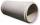Concrete pipe is cylindrical with an inner diameter 110 cm and outer 120 cm. Calculate the surface of the concrete pipe, if it is 9 m long.
• GreenhouseGarden plastic greenhouse is shaped half cylinder with a diameter of 6 m and base length 20 m. At least how many m2 of plastic is need to its cover?
• Cylinder heightCalculate the height of the cylinder and its surface is 2500 dm2 and the bases have a diameter 5dm.
• Fountain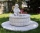The stone fountain, which has the shape of a cylinder with a diameter of 3 m, is 70 cm deep. How many m2 of stone is wetted with water?
• Roller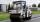Roller has a diameter of 0.96 m and a width 169 cm. How many m2 of road level when he turns 42-times?